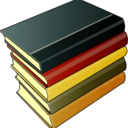# No Bullshit Guide to Linear Algebra

\$29Ivan Savov
27 ratings

Linear algebra is the foundation of science and engineering. Knowledge of linear algebra is a prerequisite for studying statistics, machine learning, computer graphics, signal processing, chemistry, economics, quantum mechanics, and countless other applications. Indeed, linear algebra offers a powerful toolbox for modelling the real world.

The No Bullshit Guide to Linear Algebra shows the connections between the computational techniques of linear algebra, their geometric interpretations, and the theoretical foundations. This university-level textbook contains lessons on linear algebra written in a style that is precise and concise. Each concept is illustrated through definitions, formulas, diagrams, explanations, and examples of real-world applications. Readers build their math superpowers by solving practice problems and learning to use the computer algebra system SymPy to speed up tedious matrix arithmetic tasks.

• Preface
• Introduction
• 1. Math fundamentals (equations, algebra, functions, geometry, trigonometry, complex, linear equations, set notation)
• 2. Intro to linear algebra (definitions, vectors, matrices, linearity)
• 3. Computational linear algebra (RREF, multiplication, determinants, inverses)
• 4. Geometric aspects of linear algebra (lines, planes, projections, vector spaces)
• 5. Linear transformations (matrix representations, change of basis for matrices, invertible matrix theorem)
• 6. Theoretical linear algebra (eigenvectors, abstract vector spaces, Gram–Schmidt, decompositions)
• 7. Applications (chemical equations, economics, circuits, graphs, least squares, computer graphics, cryptography, error-correcting codes, Fourier analysis)
• 8. Probability theory (distributions, Markov chains, PageRank)
• 9. Quantum mechanics (Dirac notation, postulates, polarizing lenses experiment, applications)
• B. Notation
• Index

"The book explains the concepts in a way that gives a strong intuitive understanding." — Joe, student

"It’s very well written and a fun read!" — Felix, professor

"I used this book in multiple big data courses when I needed a deeper understanding of the material." — Zane Zakraisek, student

"One of the best math books I've ever read and I've been reading math books for 50 years." — Mark, teacher

"This book presents the subject of linear algebra in a no nonsense way. Proofs are given that are easy to follow. Explanations are give using appropriate examples." — Claire, student

"I bought this book to get rid of certain weaknesses regarding the subject that have occurred since I left university. The book has a fine structure, symbols and functions are well defined when they are introduced. The language used is precise and easy to read, it does not loose itself in too theoretical dimensions before explaining the basics. A quality I miss in almost any book regarding higher mathematics." — Patrick, adult learner

"I highly recommend this book for beginners who need to learn linear algebra with some rigor. I used this book to study for a college math course in mechanical engineering in Germany. I found the lectures poor and taught myself most of the course content from this book to get an A. There is little fluff in this book. It built understanding and confidence. It helped me actually enjoy linear algebra. I found the writing, explanations and illustrations helpful. Particularly I like the author's approach to demystifying math by plainly stating the relationships between mathematical concepts and by breaking concepts into concrete, achievable goals. [...] the author  responds to questions, accepts feedback and continuously updates the text."  — Sean, student

"I wish text books were written so clearly. I purchased this book as a means of reviewing what i had learned in classes 30 or so years ago. This book is so well written that I not only regained what I had first learned but also learned far more and with a deeper understanding of the topic. Great book and I highly recommend it to anyone want to learn for the first time or get back up to speed on the topic." — T.D., adult learner

Sidenote: If your math skills are very rusty (e.g. you don't remember what the graph of y=mx+b looks like), you will want to read the No Bullshit Guide to Mathematics first. It  covers the same material as in Chapter 1 (review of math fundamentals), but in a less rushed manner and with more practice exercises.

You'll get the ebook and all future updates.

##### Formats
pdf (2x), epub, and mobi
780992001025
2.2
June 2021
599

5.0
(27 ratings)
5 stars
100%
4 stars
0%
3 stars
0%
2 stars
0%
1 star
0%
\$29

27 ratings